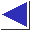# comp.lang.c FAQ list ·Question 13.16

Q: How can I get random integers in a certain range?

A: The obvious way,

```	rand() % N		/* POOR */
```
(which tries to return numbers from 0 to N-1) is poor, because the low-order bits of many random number generators are distressingly non-random. (See question 13.18.) A better method is something like
```	(int)((double)rand() / ((double)RAND_MAX + 1) * N)
```

If you'd rather not use floating point, another method is

```	rand() / (RAND_MAX / N + 1)
```
If you just need to do something with probability 1/N, you could use
```	if(rand() < (RAND_MAX+1u) / N)
```
All these methods obviously require knowing RAND_MAX (which ANSI #defines in <stdlib.h>), and assume that N is much less than RAND_MAX.

When N is close to RAND_MAX, and if the range of the random number generator is not a multiple of N (i.e. if (RAND_MAX+1) % N != 0), all of these methods break down: some outputs occur more often than others. (Using floating point does not help; the problem is that rand returns RAND_MAX+1 distinct values, which cannot always be evenly divvied up into N buckets.) If this is a problem, about the only thing you can do is to call rand multiple times, discarding certain values:

```	unsigned int x = (RAND_MAX + 1u) / N;
unsigned int y = x * N;
unsigned int r;
do {
r = rand();
} while(r >= y);
return r / x;
```

For any of these techniques, it's straightforward to shift the range, if necessary; numbers in the range [M, N] could be generated with something like

```	M + rand() / (RAND_MAX / (N - M + 1) + 1)
```

(Note, by the way, that RAND_MAX is a constant telling you what the fixed range of the C library rand function is. You cannot set RAND_MAX to some other value, and there is no way of requesting that rand return numbers in some other range.)

If you're starting with a random number generator which returns floating-point values between 0 and 1 (such as the last version of PMrand alluded to in question 13.15, or drand48 in question 13.21), all you have to do to get integers from 0 to N-1 is multiply the output of that generator by N:

```	(int)(drand48() * N)
```

References: K&R2 Sec. 7.8.7 p. 168
PCS Sec. 11 p. 172